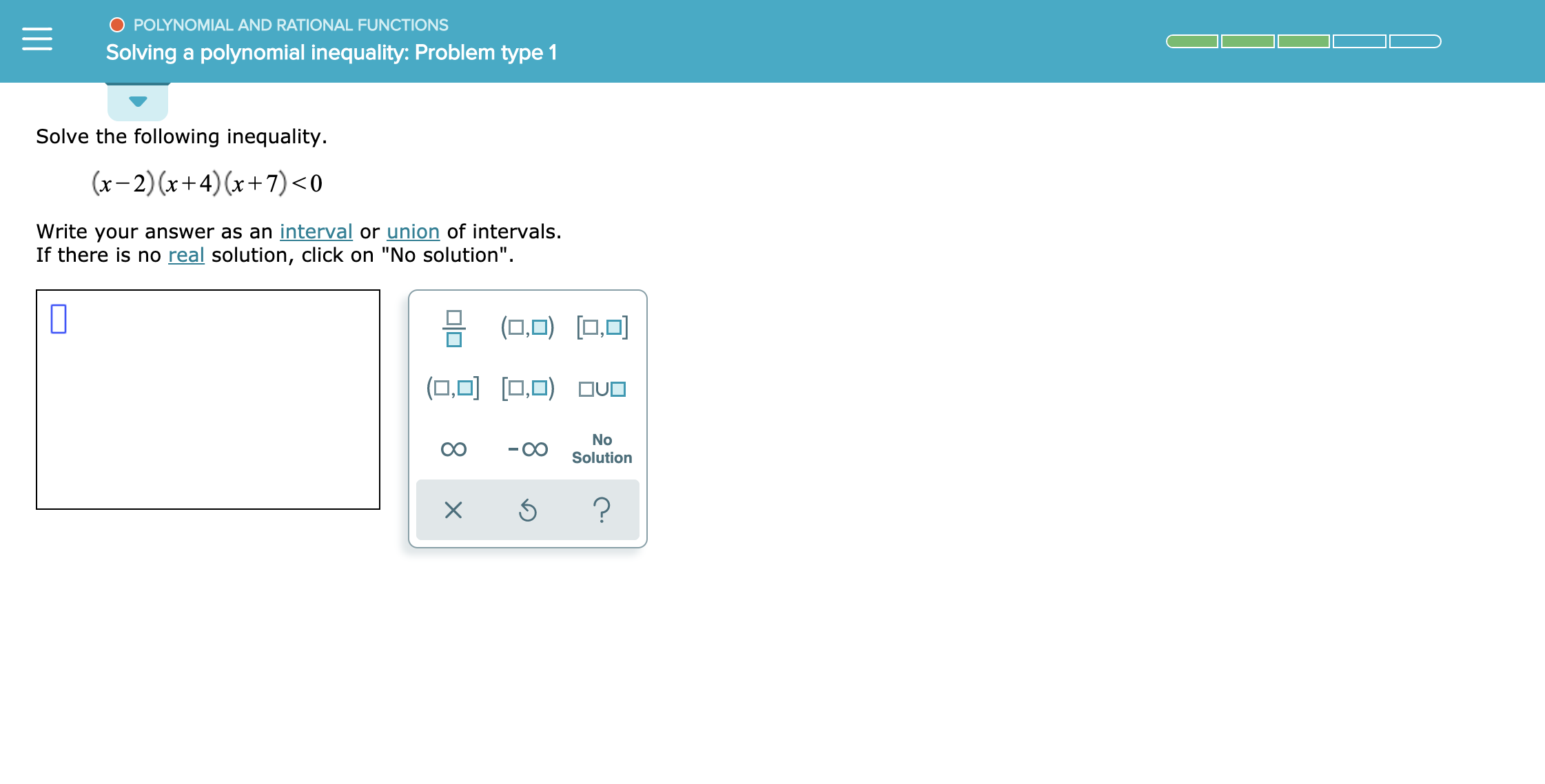# POLYNOMIAL AND RATIONAL FUNCTIONSSolving a polynomial inequality: Problem type 1Solve the following inequality.(x-2) x+4) (x+7)

Question

see attachmenthelp_outlineImage TranscriptionclosePOLYNOMIAL AND RATIONAL FUNCTIONS Solving a polynomial inequality: Problem type 1 Solve the following inequality. (x-2) x+4) (x+7)
check_circle

Step 1

The given inequality is:

Step 2

To find solution of this inequality, equate (x-2)(x+4)(x+7)=0 then

we have,

Step 3

Now, put this value of x in the real line t...

### Want to see the full answer?

See Solution

#### Want to see this answer and more?

Solutions are written by subject experts who are available 24/7. Questions are typically answered within 1 hour.*

See Solution
*Response times may vary by subject and question.
Tagged in

### Calculus